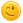# Understanding behind AF(a ∧ AXa)

Hello Professor, could you please clarify my understanding of the above formula:

I read the above as : On all paths , eventually there is 'a' and thereafter on all paths from 'a', the next state should also be 'a'. That is , in case there are multiple paths from the state that holds 'a', then all those paths should also have the next state as 'a'. If not the above statement is false.

Thanks a lot.

First of all, the portal should be a q2a system for everyone not just a professor is speaking to students, also students can communicate and discuss with other students. To that end, the users are anonymous as I am, too.You explain the formula as it is, I can't do better. Maybe just note that "paths" means always "infinite paths" in temporal logics. Also have a look at slide 38 in the temporal logic chapter, where that formula is also discussed.

by (142k points)
Thank you for the clarification. I was trying to understand the following but I am getting confused in this. How should I interpret this formula:
E((Fq) ∧ EG(p ∧ ¬q)) after looking at the answer in the following paper: https://es.cs.uni-kl.de/teaching/vrs/exams/2020.02.19.vrs/2020.02.19.vrs.solutions.pdf (page: 22)
Consider the inner formula EG(p∧¬q) first, and observe that it holds on s0,s1. Then label these states with a new variable x and consider E((Fq) ∧ x). It holds on state s0 that satisfies x and has a path where eventually q holds, namely the path from s0 to s2. Moreover, consider EF(q ∧ x) which holds in none of the states since q ∧ x holds in none of the states.